# Superconductor: models with dependence on T

## Power law with dependences on T

The critical current density Jc and the exponent n are the quantities that depend on the temperature T.

The general form of the previous function is thus the following one: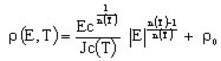(3)

where:

• Ec is the critical electric field [V/m]
• Jc(T) is the dependence on T of the critical current density (see below)
• n(T) is the dependence on T of the exponent (see below)
• ρ0 is an additional resistivity [Ω .m]

The dependence on T of the quantities Jc and n can be expressed using different models as that is presented in the tables below.

Theoretical dependence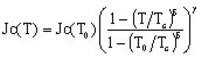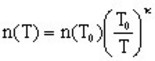5 additional parameters: T0 Tc δ γ κ
Practical dependence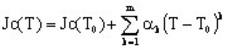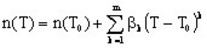2 m +1 additional parameters: T0 and αk βk